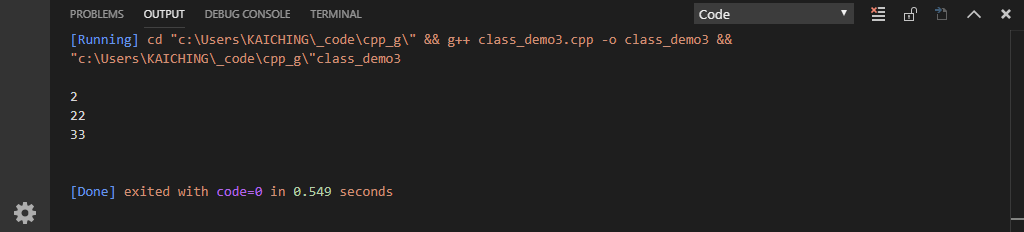# 單元 12 - 建構函數

``int a = add(12, 32);``

``double b = add(2.53, 32);``

``````double add(double p_a, int p_b) {
double c = (double) p_b;
return p_a + c;
}``````

``````Demo();
Demo(int);
Demo(int, int);``````

``````// 沒有參數的建構函數
Demo::Demo() {
set_a(1);
set_b(1);
}

// 一個參數的建構函數
Demo::Demo(int n1) {
set_a(n1);
set_b(n1);
}

// 兩個參數的建構函數
Demo::Demo(int n1, int n2) {
set_a(n1);
set_a(n1);
set_b(n2);
}``````

``````#include <iostream>

using namespace std;

class Demo {
// 宣告 public 的成員
public:
Demo();
Demo(int n1);
Demo(int n1, int n2);
void set_a(int n);
void set_b(int n);
int get_a();
int get_b();
int do_something();

// 宣告 private 的成員
private:
int a;
int b;
};

// 沒有參數的建構函數
Demo::Demo() {
set_a(1);
set_b(1);
}

// 一個參數的建構函數
Demo::Demo(int n1) {
set_a(n1);
set_b(n1);
}

// 兩個參數的建構函數
Demo::Demo(int n1, int n2) {
set_a(n1);
set_a(n1);
set_b(n2);
}

int Demo::do_something() {
// 改成呼叫 getter 成員函數
return get_a() + get_b();
}

// setter 與 getter 成員函數
void Demo::set_a(int n) {
a = n;
}

void Demo::set_b(int n) {
b = n;
}

int Demo::get_a() {
return a;
}

int Demo::get_b() {
return b;
}

int main(void) {
Demo t1;
Demo t2(11);
Demo t3(11, 22);

cout << endl;
cout << t1.do_something() << endl;
cout << t2.do_something() << endl;
cout << t3.do_something() << endl;
cout << endl;

return 0;
}

/* 《程式語言教學誌》的範例程式
http://kaiching.org/
檔名：class_demo3.cpp
功能：示範定義類別
作者：張凱慶 */``````

``````Demo t1;
Demo t2(11);
Demo t3(11, 22);``````1. 具有多個參數版本的函數被稱為多載函數，也就是允許函數有不同的參數列。
2. C++ 是強型態的程式語言，運算式中若有兩種不同的資料型態，就需要先進行型態轉換。
3. 編譯器會替基本內建型態進行自動型態轉換。
4. 函數只能有一個回傳值，若只有回傳值型態不同，這並不構成另一個多載函數。
5. 建構函數的主要目的是設定變數成員。

1. 為什麼兩個函數只有回傳值型態的不同，並不構成多載函數？
2. 為什麼編譯器要替基本內建型態進行自動型態轉換？
3. 建構函數的用途為何？除了設定變數成員外，建構函數還有其他功能嗎？

1. 寫一個程式 exercise1201.cpp ，利用 exercise1101.cpp 設計的 IntegerDemo ，加入建構函數。
2. 寫一個程式 exercise1202.cpp ，利用 exercise1102.cpp 計算階層值的類別，加入建構函數。
3. 寫一個程式 exercise1203.cpp ，利用 exercise1103.cpp 計算費氏數列的類別，加入建構函數。